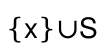6.1

Unstable Redex: May Change Without Warning

This library is unstable; compatibility will not be maintained. See Unstable: May Change Without Warning for more information.

 (require unstable/gui/redex) package: unstable-redex

This library provides functions to help typesetting for redex models. The following example program provides an overview of the features:

 > (define-language ZF [e empty (Set e) (Union e_1 e_2) (Powerset e) ZZ variable-not-otherwise-mentioned] [formula (same? e_1 e_2) (in? e_1 e_2) true false (implies formula_1 formula_2)])

By default, Redex models are typeset as S-expressions with some basic styling that distinguishes literals from nonterminal names, handles subscripting, etc.

 > (language->pict ZF)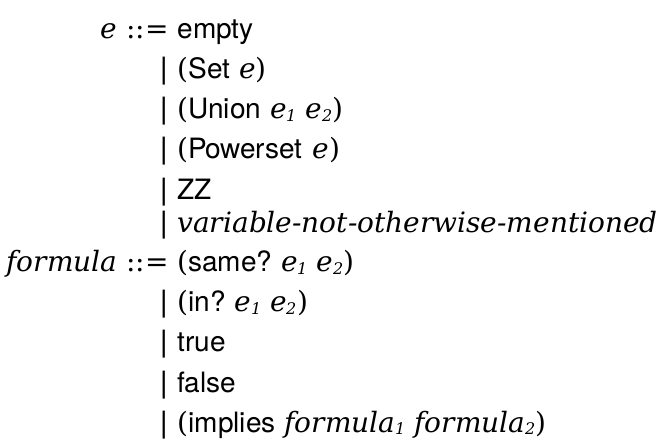> (term->pict ZF (in? x (Set 1 2 3 ...)))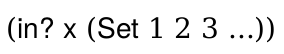This library provides helper functions for creating and using rewriters that transform the S-expression model terms into other notations.

 > (add-atomic-rewriters! 'empty "∅" 'formula "φ" 'ZZ (text "Z" '(bold . modern) (default-font-size)) 'variable-not-otherwise-mentioned (lambda () (text "x, y, z, ..." (literal-style) (default-font-size))) 'true (lambda () (text "true" '(caps . modern) (default-font-size))) 'false (lambda () (text "false" '(caps . modern) (default-font-size))))
 > (add-compound-rewriters! 'same? (binary-rw " = ") 'in? (binary-rw " ∈ ") 'Set (bracket-rw 'curly) 'Powerset (function-rw "P") 'Union (binary-rw "∪") 'implies (binary-rw " ⇒ " #:parenthesize-left '(implies)))
 > (with-rewriters (lambda () (language->pict ZF)))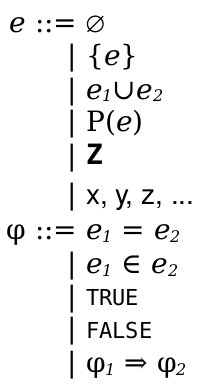> (with-rewriters (lambda () (render-term ZF (in? x (Set 1 2 3 ...)))))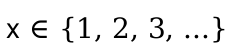procedure(with-rewriters proc) → any proc : (-> any)

parameter

 (let ([atomic-rewriter/c (or/c string? pict? (-> (or/c string? pict?)))]) (plistof symbol? atomic-rewriter/c))
(current-atomic-rewriters rewriters)  void?
rewriters :
 (let ([atomic-rewriter/c (or/c string? pict? (-> (or/c string? pict?)))]) (plistof symbol? atomic-rewriter/c))
Parameter of atomic rewriters (as in with-atomic-rewriter) used by with-rewriters.

 parameter → (plistof symbol? compound-rewriter/c) (current-compound-rewriters rewriters) → void? rewriters : (plistof symbol? compound-rewriter/c)
Parameter of compound rewriters (as in with-compound-rewriter) used by with-rewriters.

 parameter → (plistof (-> lw? any/c) (-> lw? lw?)) (current-unquote-rewriters rewriters) → void? rewriters : (plistof (-> lw? any/c) (-> lw? lw?))
Parameter of unquote rewriters (as in with-unquote-rewriter) used by with-rewriters.

procedure

rewriters :
 (let ([atomic-rewriter/c (or/c string? pict? (-> (or/c string? pict?)))]) (plistof symbol? atomic-rewriter/c))
 procedure(add-compound-rewriters! rewriters) → void? rewriters : (plistof symbol? compound-rewriter/c)
 procedure(add-unquote-rewriters! rewriters) → void? rewriters : (plistof (-> lw? any/c) (-> lw? lw?))

 procedure(plistof key/c value/c) → contract? key/c : contract? value/c : contract?
Contract for even-length lists of alternating key/c and value/c values.

Equivalent to
 (letrec ([ctc (recursive-contract (or/c '() (cons/c key/c (cons/c value/c ctc))))]) ctc)

 value
Contract for compound rewriters, which take a list of lw structs and returns a list of lws, picts, or strings.

Equivalent to
 (-> (listof lw?) (listof (or/c lw? pict? string?)))

procedure

 (binary-rw operator [ #:parenthesize-arg parenthesize-arg #:parenthesize-left parenthesize-left #:parenthesize-right parenthesize-right])
compound-rewriter/c
operator : (or/c string? pict? (-> (or/c string? pict?)))
 parenthesize-arg : (or/c #t #f (listof symbol?) (-> lw? any/c)) = #f
 parenthesize-left : (or/c #t #f (listof symbol?) (-> lw? any/c)) = parenthesize-arg
 parenthesize-right : (or/c #t #f (listof symbol?) (-> lw? any/c)) = parenthesize-arg
Typesets (sym term1 term2) using operator as a binary operator between term1 and term2.

Examples:

 > (add-compound-rewriters! 'plus (binary-rw " + "))
 > (with-rewriters (lambda () (term->pict ZF (plus 1 2))))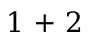Redex terms may become ambiguous when typeset. To avoid ambiguity, use #:parenthesize-arg to direct when arguments should be parenthesized. If parenthesize-arg is #t, then arguments are always parenthesized; if it is #f, never; if it is a list of symbols, then an argument is parenthesized only if the argument is a term starting with a symbol in the list; if it is a procedure, then the argument is parenthesized if the procedure applied to the argument’s lw struct returns a true value.

 > (add-compound-rewriters! 'times (binary-rw " × "))
 > (with-rewriters (lambda () (term->pict ZF (times (plus 1 2) 3))))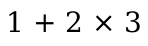> (add-compound-rewriters! 'times (binary-rw " × " #:parenthesize-arg '(plus)))
 > (with-rewriters (lambda () (term->pict ZF (times (plus 1 2) 3))))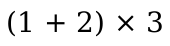The parenthesization rules for left and right arguments can be supplied separately through #:parenthesize-left and #:parenthesize-right, for example to create left-associated or right-associated operators:

 > (add-compound-rewriters! 'arrow (binary-rw " → " #:parenthesize-left '(arrow)))
 > (with-rewriters (lambda () (term->pict ZF (arrow (arrow A B) (arrow C D)))))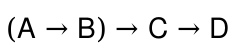procedure

 (prefix-rw prefix [ #:parenthesize-arg parenthesize-arg])
compound-rewriter/c
prefix : (or/c string? pict? (-> (or/c string? pict?)))
 parenthesize-arg : (or/c #f #t (listof symbol?) (-> lw? any/c)) = #f
Typesets (sym term) by placing prefix before term.

Examples:

 > (add-compound-rewriters! 'not (prefix-rw "¬ "))
 > (with-rewriters (lambda () (term->pict ZF (not (in? x empty)))))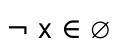procedure

 (postfix-rw postfix [ #:parenthesize-arg parenthesize-arg])
compound-rewriter/c
postfix : (or/c string? pict? (-> (or/c string? pict?)))
 parenthesize-arg : (or/c #f #t (listof symbol?) (-> lw? any/c)) = #f
Typesets (sym term) by placing postfix after term.

Examples:

 > (add-compound-rewriters! 'nonempty (postfix-rw " is nonempty"))
 > (with-rewriters (lambda () (term->pict ZF (nonempty (Set x)))))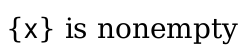procedure(function-rw function) → compound-rewriter/c function : (or/c string? pict? (-> (or/c string? pict?)))
Typesets (sym term ...) by placing function before the parenthesized, comma-separated list of terms.

Examples:

 > (add-compound-rewriters! 'f (function-rw "f") 'max (function-rw (text "max" '(bold . modern) (default-font-size))))
 > (with-rewriters (lambda () (term->pict ZF (max 1 2 (f 3)))))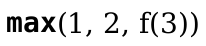procedure
Typesets (sym term1 term2 ...) as term1. Useful for hiding parameters that are necessary for defining the semantics but can be glossed over in its explanation, such as state parameters used for generating unique names.

Examples:

 > (add-compound-rewriters! 'First (only-first-rw))
 > (with-rewriters (lambda () (term->pict ZF [First (in? x y) counter])))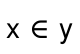procedure
Typesets (sym term ...) by rendering the terms side-by-side.

 procedure(constant-rw constant) → compound-rewriter/c constant : (or/c string? pict? (-> (or/c string? pict?)))
Typesets (sym term ...) as constant.

procedure

(bracket-rw brackets [#:comma? comma?])  compound-rewriter/c

brackets :
 (or/c 'round 'square 'curly 'angle (list (or/c string? pict?) (or/c string? pict?)))
comma? : any/c = #t
Typesets (sym term ...) by surrounding the comma-separated (or space-separated, if comma? is false) sequence of terms with brackets. If brackets is a list, the first element is the left bracket and the second is the right bracket; 'round is equivalent to '("(" ")"); 'square is equivalent to '("[" "]"); 'curly is equivalent to '("{" "}"); and 'angle is equivalent to '("〈" "〉").

Examples:

 > (add-compound-rewriters! 'Tuple (bracket-rw 'angle))
 > (with-rewriters (lambda () (term->pict ZF (Tuple 1 2 3))))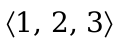procedure
Rewriter that typesets (sym elem-term set-term) as the union of the singleton set containing elem-term with the set set-term.

Examples:

 > (add-compound-rewriters! 'set-cons (set-cons-rw))
 > (with-rewriters (lambda () (term->pict ZF (set-cons x S))))